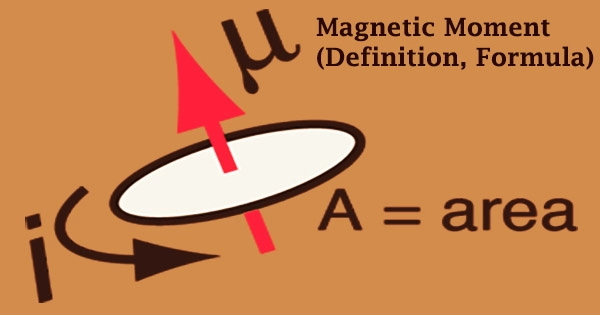Physics

# Magnetic Moment (Definition, Formula)The magnetic moment, sometimes called the magnetic dipole moment, is a feature of a magnet that interacts with an applied field to produce a mechanical moment. Loops of electric current (such as electromagnets), permanent magnets, elementary particles (such as electrons), different chemicals, and numerous astronomical objects are examples of things with magnetic moments (such as many planets, some moons, stars, etc). The magnetic moment is a vector quantity, and things seek to align themselves such that the magnetic moment vector is parallel to the magnetic field lines.

A magnet’s magnetic moment is a number that defines the force that it may exert on electric currents as well as the torque that a magnetic field will apply on it. The component of the magnetic moment may be represented by an analogous magnetic dipole, which consists of a magnetic north and south pole separated by a little distance. Magnetic moments may be found in a loop of electric current, a bar magnet, an electron (revolving around a nucleus), a molecule, and a planet.

The magnetic moment of a magnet is measured from the south pole to the north pole. For tiny enough magnets or long enough distances, it is adequate. For extended objects, higher-order components (such as the magnetic quadrupole moment) may be required in addition to the dipole moment. A magnet’s magnetic field is precisely proportional to its magnetic moment. The amplitude (and direction) of this torque is determined by the magnetic moment’s magnitude as well as its orientation in relation to the magnetic field’s direction.

The susceptibilities of magnetic materials can be classed as diamagnetic, paramagnetic, or ferromagnetic. Magnetic moment and magnetic field may both be thought of as vectors with magnitude and direction. A magnetic dipole’s magnetic field is proportional to the magnetic dipole moment of the dipole. The dipole component of an object’s magnetic field is symmetric along the direction of its magnetic dipole moment and diminishes as the inverse cube of the object’s distance.

The magnetic moment is directed from the south pole to the north pole of the magnet. The magnet’s magnetic moment is proportional to the magnetic field it produces. The magnetic moment is a vector that connects the aligning torque on an item caused by an external magnetic field to the field vector itself. The relationship is given by:

τ = m × B

where τ is the torque acting on the dipole, B is the external magnetic field, and m is the magnetic moment. A magnet’s magnetic field is proportional to its magnetic moment, as well. The phrase magnetic moment usually refers to a system’s magnetic dipole moment, which forms the first component in a general magnetic field’s multipole expansion. Magnetic moment is generated by the following two methods:

• The motion of Electric Charge
• Spin Angular Momentum

The mechanical angular momentum is connected to the magnetic moment. The gyromagnetic ratio is the ratio of magnetic moment to angular momentum. The intrinsic energy of a system, in general, encompasses the system’s self-field energy as well as the energy of the system’s internal workings. Magnetometers are equipment that are used to measure magnetic moments. However, not all magnetometers are positioned to directly detect the magnetic moment.

Some of these sensors just measure magnetic fields, and the magnetic moment is calculated from the observed magnetic field. The orbital angular momentum of atoms is quantized, and so magnetic moment components are quantized as well. Only the components of the spin magnetic moment along the direction of the magnetic field may be measured directly.

The orbital angular momentum of atoms is quantized, and so magnetic moment components are quantized as well. Only the components of the spin magnetic moment along the direction of the magnetic field may be measured directly. In the International System of Units (SI) base units, the unit for a magnetic moment is A⋅m2, where A is ampere (SI base unit of current) and m is meter (SI base unit of distance).

When domains of elementary moments are modified to have some magnetic moment as a whole, ferromagnetic substances gain magnetic moment. If m is the magnetic pole’s power, the magnetic dipole moment of the magnet is represented by the vector M, which is written as

M = m / 2 ι

Magnetometers are commonly used to measure the magnetic moments of objects, albeit not all magnetometers measure magnetic moments: Instead, some are set up to measure magnetic fields. The magnetic moment of subatomic particles does not have a fixed direction, but it does have quantized orientations in the presence of a magnetic field with respect to the direction of the applied magnetic field (space quantization).

Information Sources: